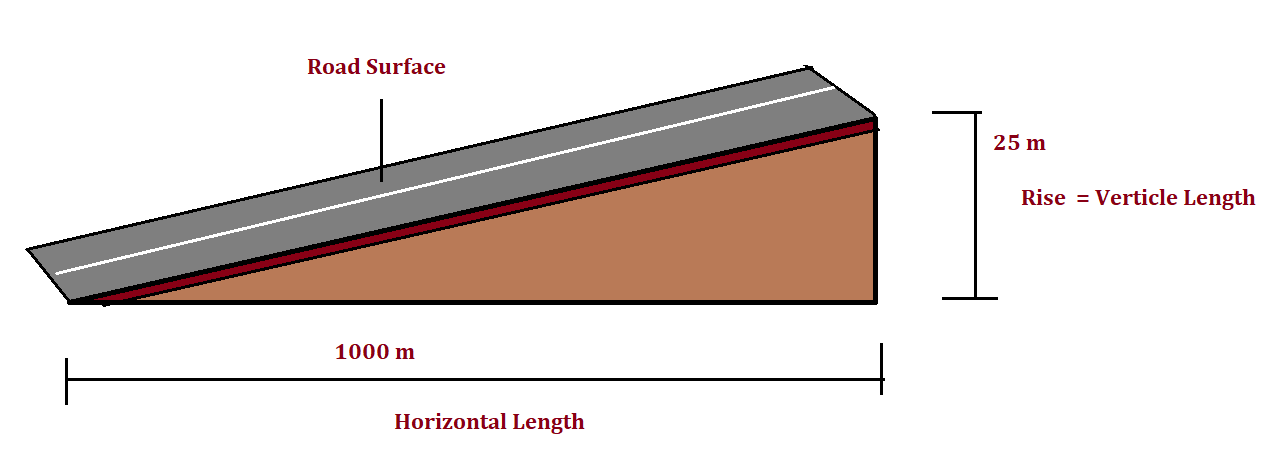16th September 2017

The rate of rise or fall along the length of the road with respect to the horizontal is called grade or gradient.

In other words, it is the longitudinal slope provided to the formation level of a road along its alignment. It is expressed as the ratio of 1 in n (1 vertical unit to n horizontal units). It is also expressed as a percentage.

The gradient may also be expressed in degrees of elevation or depression above or below the horizontal plane. Mathematically, the gradient can be expressed as follows:
or
gradient =  (1/n) * 100 Percent.

Lets, take an example,

In the above picture, the horizontal distance is 1000 m, and verticle distance or rise or fall is 25 m. From the definition, we can write,
Gradient = 25 : 1000 = 1 : 40.
Or, 1 in 40.,
Or, (1/40) * 100 = 2.5 percent